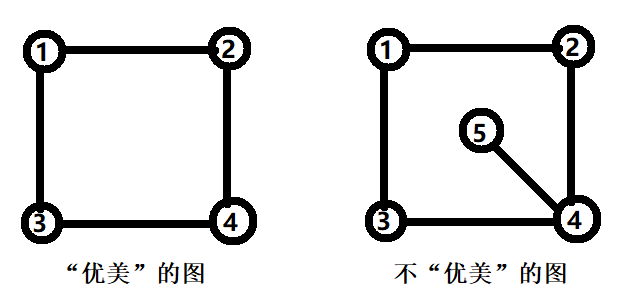# #632. 【UR #21】挑战最大团### 样例一

#### input

4
5
1
1



#### output

4 4 0 0



#### explanation

0101
1010
0101
1010


### 样例二

#### input

10
FF1
FF
F7
F3
F1
F
7
3
1



#### output

10 45 120 210 252 210 120 45 10 1



### 数据范围

$1$ $20$ $15$
$2$ $1000$ $m=\frac{n(n-1)}{2}$ $5$
$3$ A $10$
$4$ $m\geq \frac{n(n-1)}{2}-20$ $10$
$5$ $20$
$6$ $4000$ $10$
$7$ $8000$ $30$